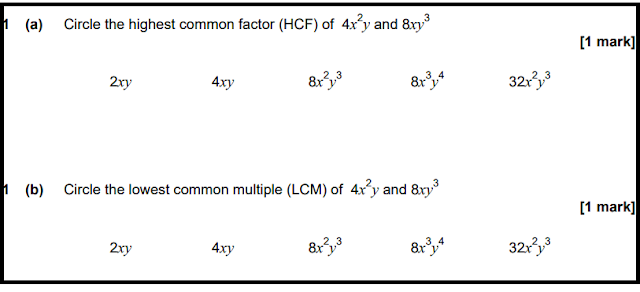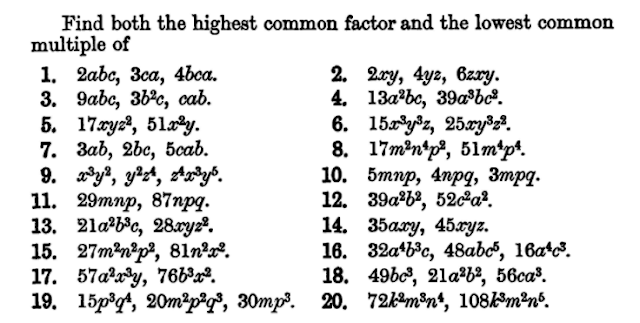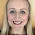## 30 May 2018

### Algebraic HCF and LCM

The topic 'Highest Common Factor and Lowest Common Multiple' is one where there's a lot of scope for subject knowledge development. There is so much for both teachers and students to explore in this topic. Ask a group of maths teachers how they find the highest common factor of two numbers and I expect a number of different methods will be mentioned. There is no universally accepted 'best way'. I wrote about a number of alternative approaches back in 2015.

Identifying Highest Common Factors
When I think of highest common factor questions, I think of questions involving integers. For some reason I've never taught a lesson on 'Highest Common Factor of Algebraic Terms'. On reflection, I think it might really help develop student understanding of the underlying concepts.

Look at these two examples:

Find the Highest Common Factor of 420 and 96
Find the Highest Common Factor of 10ab and 5a2b2

In the first case we want to find the greatest integer that is a factor of both 420 and 96. The most conceptually clear approach is to list all the factors of 420 and all the factors of 96 and then find the biggest factor that's on both lists. But it can take a while to list all the factors, so we often use primes to arrive at the answer more quickly. We write

420 = 2 x 2 x 3 x 5 x 7
96 = 2 x 2 x 2 x 2 x 2 x 3

Many students then use a Venn method to identify the highest common factor. I suspect that although students can follow the Venn procedure fairly easily, they may not really know what's going on. When teaching this from first principles, it's helpful to rewrite the factors like this

420 = 2 x 2 x 3 x 5 x 7 = 12 x 35
96 = 2 x 2 x 3 x 2 x 2 x 2 = 12 x 8

And then it's so much clearer why 12 is the highest common factor.

The extract below is taken from 'New General Mathematics' (first published in 1956 and still in use in the 1980s). We can see the conceptual understanding being embedded much more carefully than it is now. Whereas modern textbooks often jump straight to questions like 'find the HCF and LCM of 45 and 60', which requires a number of separate skills, here we can see isolation of the specific skill 'identify the common factors'. This seems a far more sensible starting point from a cognitive load perspective.

Students are not required to do the prime factorisation first, nor are they being asked to find both a HCF and LCM. They are simply selecting the common prime factors. In the first question, all three numbers contain 3 x 3, meaning they are all multiples of 9. There is no other factor that appears in all three numbers, so 9 is the highest common factor. Later in the exercise students will need to do the prime factorisation themselves, but not yet.
Of course, the idea is the same for the algebraic terms. But it's easier, because it's immediately clear what the algebraic factors are:

10ab3 = 2 x 5 x a x b x b x b = 5ab2 x 2b
5a2b= 5 x a x a x b x b 5ab2 x a

For numbers, finding a highest common factor can take a bit of work - it's harder to identify the factors of a number than the factors of an algebraic term. But the underlying mathematical concept is identical. Perhaps it might even be better to start with algebra before moving onto numbers.

Factorising with Highest Common Factors
Factorising an algebraic expression by identifying the highest common factor of two or more terms is relatively easy once you understand how index notation works.

10ab+ 5a2b2  = 5ab2(2b + a)

We don't often factorise in the same way with numbers, but it's fun to play with:

Factorise 56 + 42

Here we take out the highest common factor, giving us 14(4 + 3). And this is nice, because we can now see that 56 + 42 is the same as 14 x 7. That's because it's four lots of 14 plus three lots of 14.

Here's another one:

Factorise 24 + 36

Here we take out the highest common factor of 12, giving us 12(2 + 3). So we can now see that 24 + 36 is the same as 12 x 5. Which is obvious when you think about it, because it's (12 + 12) + (12 + 12 + 12). It's fun to play with numbers like this.

There are plenty of fun numerical factorisation questions in textbooks, old and new.
Identifying Lowest Common Multiples
Identifying lowest common multiples using primes can be quite conceptually challenging. Students can be successful when using Venn methods and similar, but I bet few would be able to explain how their method works.

I like this introduction from a 1950s textbook for starting to build an understanding of what's going on:

What factors must necessarily be in a number so that 2, 5 and 7 will divide into it exactly?

What factors must necessarily be in a number so that 3, 2 x 3 and 3 x 5 will divide into it exactly?

What factors must necessarily be in a number so that 4x, 2ax and 6a will divide into it exactly?

I think it might be worth developing this as a starting point when introducing this topic.

At primary school, students learn to add and subtract fractions using lowest common denominators, but they may not realise that in doing so they are actually finding LCMs. I wonder if we could make better links to this prior knowledge.

Questions for Developing Fluency
This question came up in an AQA mock last year:AQA GCSE Practice Set 4 (Paper 1H)

When marking this I noticed that although I hadn't covered it directly with my students, most were able to work it out using their understanding of HCF and LCM.

Later I stumbled upon a huge set of questions just like this! The extract below is from Elementary Algebra for Schools, a textbook from the late 1800s that has been fully digitised.It makes a lot of sense to develop fluency here, before moving onto related skills such as factorisation into single brackets and simplifying algebraic fractions.

The book takes this concept a lot further, looking at LCMs and HCFs of compound expressions including quadratics and cubics.
This chapter on algebraic lowest common multiples comes before the chapter on 'Adding and Subtracting Algebraic Fractions'. This makes a lot of sense!

When I teach adding algebraic fractions I don't directly teach the separate skill of finding the LCM of algebraic expressions beforehand. Perhaps I should. To add or subtract algebraic fractions like those shown below, there are a number of key skills:
1. identifying the lowest common multiple of the denominators
2. converting fractions so they share a denominator
3. adding the numerators once the fractions have the same denominator
4. simplifying
There's a lot going on here. We need to take these skills one at a time.
There are dozens more pages on algebraic highest common factor and lowest common multiple in this Victorian textbook. It was clearly a big part of secondary school mathematics in the 1800s.

There are similar activities in textbooks from the 1950s. They contain loads of practice on HCF and LCM of algebraic terms and expressions.
In stark contrast, there are only a handful of relatively simple questions in a modern GCSE textbook on the same skill:

It's funny how things change.

I hope you've found this useful, or if not useful then at least interesting! If you have anything to share regarding algebraic highest common factor and lowest common multiple, please tweet me or comment below. Thanks for reading.

1.I like your point that perhaps we should use letters first before numbers. If students can work with the abstract then the pattern is so much more evident. Great examples from older textbooks - Hall and Knight's Elementary Algebra new to me!

1.Thank you!

2.Hey Jo. Brilliant! I did loads mental arithmetic age 6-7, I used Lacombe and New General Maths books throughout Primary and Grammar(not in the UK selective sense). I resonate with apt attention paid to subtle and latent links in older textbooks. Therein lies the rigour. We moved away from it and introduced an ever widening gap of Misconceptions. We also since then started seeing procedural as enemy of conceptual. In the not too distant past concept was thought rigorously then once grasped procedure made maths fun. Glad we are finding the light again. If you find a place were I can acquire the above two textbooks for all year groups please let me know asap. Cheers.

1.Thanks for the comment! Absolutely agree.

2.3.Hi Jo. Great website. Just wondering whether there are any answers for this page?
Thanks

1.For which exercise? The exercises in this post come from a number of sources. Some have answers, some don't.